# SSC Quantitative Aptitude Questions (Day-47)

Dear Aspirants, you can find the Quantitative Aptitude questions with detailed explanations for the SSC exams. Nowadays the competitive level of the exam has been increasing consistently. Due to the great demand for the government job, the level of the toughness reached greater. Candidates have to enhance the preparation process in order to drive in the right path. It doesn’t need to clear the prescribed cutoff. You must have to score good marks more than the cut off marks to get into the final provisional list. Here we have updating the Quantitative Aptitude questions with detailed explanations on a daily basis. You can practice with us and measure your level of preparation. According to that you can sculpt yourself in a proper way. SSC aspirants kindly make use of it and grab your success in your career.

Start Quiz

1) What is the value of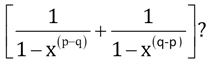(a) 0

(b) 1

(c) (xq – xp)/(xq + xp)

(d) (xq + xp)/(xq – xp)

2) If x2 – 3x + 1 = 0, then what is the value of x+
1/x?

(a) 3

(b) 7

(c) 9

(d) 11

3) If a + b + c = – 11, then what is the value of (a + 4)3 + (b + 5)3 + (c + 2)3 – 3(a + 4)(b + 5)(c + 2)?

(a) -1331

(b) -121

(c) 0

(d) 1331

4)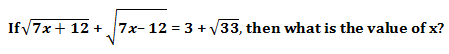(a) 0

(b) 1

(c) 3

(d) 9

5) If x + (1/x) = 3√2, then what is the value of x5 + (1/x5)?

(a) 178√3

(b) 789√2

(c) 1581√2

(d) 717√2

6) In ΔPQR, a line parallel to side QR cuts the side PQ and PR at points M and N respectively and point M divide PQ in the ratio of 1 : 2. If area of ΔPQR is 360 cm2, then what is the area (in cm2) of quadrilateral MNRQ?

(a) 160

(b) 320

(c) 120

(d) 96

7) ABC is an isosceles triangle such that AB = AC = 30 cm and BC = 48 cm. AD is a median to base BC. What is the length (in cm) of AD?

(a) 18

(b) 20

(c) 24

(d) 32

8) In ΔABC,
C = 54°, the perpendicular bisector of AB at D meets BC at E. If EAC = 42°, then what is the value (in degrees) of ABC?

(a) 25

(b) 42

(c) 50

(d) 60

9) In an isosceles triangle DEF,
D = 110°. If I is the incentre of the triangle, then what is the value (in degrees) of EIF?

(a) 110

(b) 130

(c) 145

(d) 155

10) In the following figure, O is the centre of the circle and
PRQ = 50o. What is the value (in degrees) of PTQ?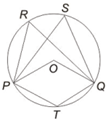(a) 100

(b) 75

(c) 130

(d) 150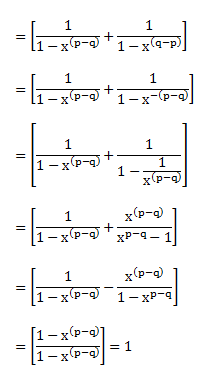x² – 3x + 1 = 0

Multiply the equation by 1/x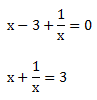a + b + c = -11

Or (a + 4) + (b + 5) + (c + 2) = 0

We know in  x³ + y³ + z³ – 3xyz = (x + y + z) (x² + y² + z² – xy – yz – zx]

If x + y + z = 0

Then, x³ + y³ + z³ – 3xyz = 0

So, the value of given equation is 0.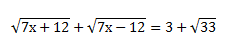By putting the value from options one by one, only

x = 3 satisfy the given equation.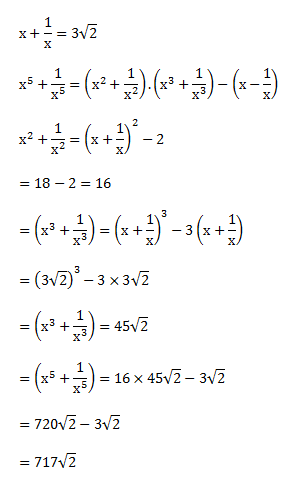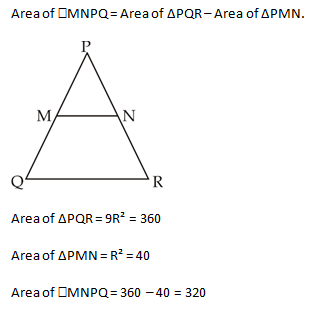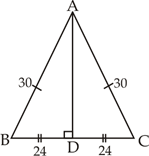Note =In isosceles triangle median is also the altitude.

So, AD² = AB² – BD² (by Pythagoras theorem)

= 30² – 24²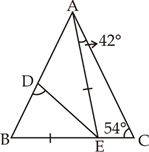In ∆AEC ; ∠AEC = 180° – (54 + 42)

= 84°

By exterior angle property

∠BAE + ∠ABE = 84° –                       …(i)

In ∆ABE,

For perpendicular bisector, AE = BE

So, ∠ABE = ∠BAE = x

By Equation (i)

2x = 84°

x = 42°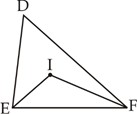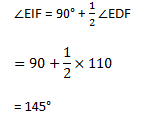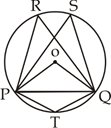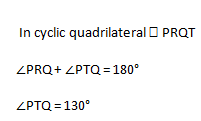Check Here to View SSC CGL / CHSL 2021 Quantitative Aptitude Questions Day – 46 Day – 45 Day – 44
5 1 vote
Rating